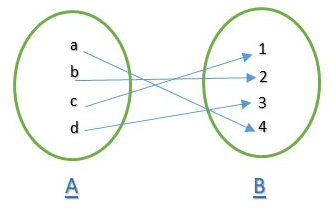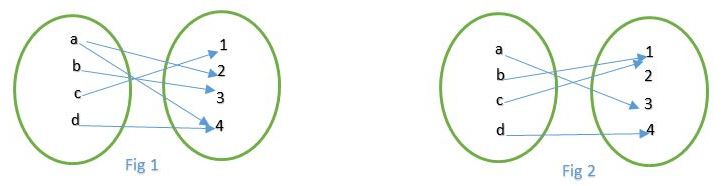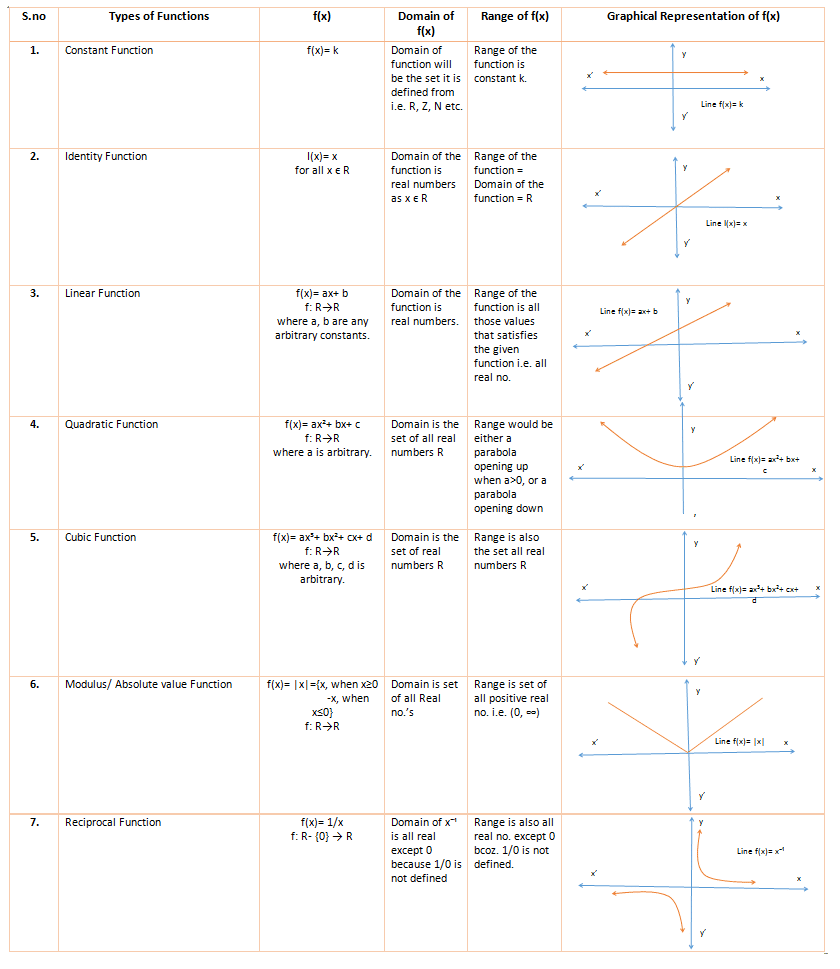# Quantitative Aptitude: Basic Concept of FunctionsLast Updated on Nov 2, 2020

“Functions can be really DECEPTIVE!!”. Yes, it is true. Many a time aspirants do get stuck.  How to start,? How to perceive?  These are the FAQ when it comes to Functions and its application. The hurdles can be categorized as  Trigonometric Functions, Algebraic functions, Modulus functions, Even/Odd, etc.

A function is a rule that relates how one quantity depends on other quantities. Many quantities depend on one or more changing variables. For example:

1. Plant growth depends on sunlight and rainfall.
2. Speed depends on distance traveled and time taken.
3. Voltage depends on current and resistance
4. Test marks depend on attitude, listening in lectures and doing tutorials (among many other variables!!)

Before directly jumping on to the types and examples of Functions first understand what they truly are which will help us understand the interrelated concepts and topics and will make solving questions easy. So, let’s begin with the Formal definition of Functions.

Let A and B be two non-empty subsets. A relation f from A to B i.e. a subset of A x B is called a function from A to B, If

1. For each, a ϵ A there exists b ϵ B such that (a, b) ϵ f.
2. (a, b) ϵ f and (a, c) ϵ f => b = c.

And that was the mathematical definition of Function now let’s learn what it means. In simple terms, if we have two set supposedly, for exampleAs given in above figure let there’s set A = {a, b, c, d} and set B = {1, 2, 3, 4} and let us call the set A be domain and B be codomain. Then, for each element present in A i.e. a, b, c, d there is a unique element corresponding to each of them in B. As you can see in the figure a goes to 4, b goes to 2, c goes to 1, and d goes to 3 i.e. for every element in domain there exist a unique element in codomain.Now, which of the above is a Function?

Fig 1 and Fig 3 are not functions (Why?). The answer lies in its definition. Therefore, Fig 1 is not a function reason being that in its Domain a has two images 2 and 4 which is not possible because as given in definition every element in its domain should have a unique image but here this is not the case. Similarly, Fig 2 is not a function with the same reason that here c in the domain has got no image in the codomain.

And, Fig 2 is a function as it satisfies the criteria of being a function that every element here in Fig 2 has got a unique image in its codomain.

### Domain and range of a function:

The set of all the inputs for a given function is called the domain of a function or if f: R→Z, here f is defined from real numbers to integer values. Then here the set of real numbers is a domain of a function. Here the set of integers Z is known as codomain.  Similarly, all the set of outputs (dependent variable) we get by plugging into the set of all inputs ( domain) in a given function is known as the range of a function.

Before moving on to questions that determine the domain and range of a function. Look at this table given below.### Summary

In this blog post, we have explained the basics of Functions with simple diagrams. Also. Domain and  Range of Function is explained through a table. By understanding the concept clearly you can make a strong hold over Quantitative Aptitude.